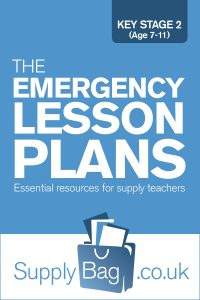# Year 5 Emergency Lesson Plans for NumeracyThese emergency lesson plans for Year 5 Numeracy are only to be used by supply teachers who are faced with no planning or easily accessible resources. Also to be used following the guidance notes here.

#### Block A – Counting, partitioning and calculating

• Using a calculator if you can find them/enough. Mental methods of adding 9, 19, 11, 31. Written methods of adding/subtracting decimals (use calculator to check only, or estimate mentally, use calculator, then check using written methods.)
• Ordering, partitioning and rounding decimals. Find decimals by first dividing numbers on a calculator by non-factors.
• Story board, one-two-step problems involving measures (Noah's Ark? weights of food needed, distance travelled in a day etc.)
• Look at 11 x and 12 x table, notice any patterns, past 100 too?
• Written methods (grid/Napier's bones? Russian?) of HTU x U, U.t x U and/or HUTE / U.

#### Block B – Securing number facts, understanding shape

• Addition and subtraction of decimals (use money – they relate well to it usually!)
• Mental recall of sums/differences/double and halves (of decimals too)
• Look at common multiples of various factors. Translate to LCM in fractions if able, 1/3 and 1/4 can be converted to 4/12 and 3/12, show physically cake/bar of chocolate cutting on board.
• Sort a set of data using a Venn Diagram, and/or a Carroll Diagram, be careful not to confuse the two.
• Using a calculator to check sums you have written on board (write ten on board, using brackets? and get about half wrong!) Children to estimate first.

#### Block C – Handling data and measures

• Probability: Children to make up predictions, it will rain tomorrow, England will win the World Cup, a dodo will be found wandering on the beach down the road, and place them on a scale of probability… use language first, never, unlikely, even chance, likely, certain, but then divide numerically, 0% chance – 100% chance.
• Find the mode: most often! Collect data from classroom, can be words (favourite author, science topic) to start, but then onto figures (month 1-12 in which born, house number etc.) Represent in frequency table, pictogram, bar/line graph and find the mode.
• Give children a set of data, hidden in story perhaps, or include irrelevant information at least, and ask them to interpret/organise into chart/table form, ready to answer your questions (the difference, the range, total, how many more if, etc.) then ask them to create two questions to ask rest of class.
• Estimating length of objects around the classroom, or go and collect a few different leaves, and estimate their lengths (place in length order first). Measure in cm.
• Give set of measurements (relate to children's lives) and ask to convert to another unit of measure, i.e. shoe 18.9cm, to mm, can of Coke 330ml, to l.

#### Block D – Calculating, measuring and understanding shape

• 24-hour clock: introduce, show how to convert, then convert meaningful times to the children (waking, start of school, etc.)
• Give children a number sentence, in which you have included brackets, show how they must calculate the brackets first, set out clearly, and ask them to work through more examples.
• Parallel and perpendicular: investigate/draw/classify shapes according to these newly learnt terms.
• Have you got protractors? Estimating, measuring and drawing given angles.
• Angles on a straight line… cut the line with a perpendicular on, then investigate lines cutting it at different angles (will have 4 angles, 2 pairs.)
• Coordinates: draw a map (theme park? treasure island? perfect school?) on the board, label the lines, not the spaces, slowly introduce coordinates in the spaces (2.5, 3) or (25, 30).
• Pattern in translation: cut a square, cut a piece out of it, and place it on the parallel side, translate shape. Children to create their own.
• Pattern in reflection: mirrors? Use hypothetical line of symmetry, reflect below, can they also reflect their simple shape to left and to right?
• Areas and/or perimeters of regular/irregular polygons, start with quadrilaterals, move onto two rectangles making an L shape.

#### Block E – Securing number facts, relationships and calculating

• Written/mental methods of up to HTU / U and TU.t * U
• Number machine: firstly give input and operation (6 goes in, machine *2, children to answer 12 is the output) change operation, then give input, and output, children to work through all 4 operations finding possible functions. Explain to children concept of scales on maps, draw simple representation on board, children use function machine idea to generate actual distances on your scaled map.
• Equivalent fractions, fractions of cake/pizza/egg boxes that are equivalent, look at patterns in equivalent fractions 'number line' and relation to times tables i.e. 1/2, 2/4, 3/6, 4/8. This has been done earlier in KS1, so quickly move onto non-unit fractions, 3/4 = 6/8 etc.
• Percentages: first introduction to percentages maybe, so look at meaning (% is out of 100, recognise division line in symbol %) 100% as whole, 50% as half, 25% and 75%. Look at how to find simple percentages of shapes to begin with, then numbers/quantities if they've got it, esp. % mentioned above, and moving onto 10%, then multiply by 4 to find 40% etc.
• Investigate connections between fractions and percentages, 1/2 of 360 = 50% of 360, etc. You write fraction question, they write equivalent percentage question etc. Then challenge them to work them out.
• 1 and 2 step word problems including decimals (use money, they relate well to it!)